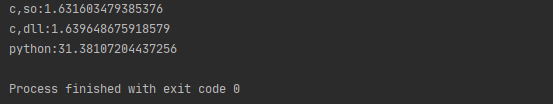## Python调用C语言

Windows下Demo亲测一波，话不多说，上图上代码。
foo.c:

``````# include<stdio.h>

int foo(){
int i,k,m;
for(i=0;i<1000;i++){
for(k=0;k<1000;k++){
for(m=0;m<1000;m++)	{
}
}
}
return 0;
}
``````

python代码

``````from ctypes import cdll
from time import time

#.c-->.so
start=time()
dll.foo()
print(f'c,so:{time()-start}')

#.c-->.dll
start=time()
dll.foo()
print(f'c,dll:{time()-start}')

#Python
start=time()
for i in  range(1000):
for k in range(1000):
for m in range(1000):
pass
print(f'python:{time()-start}')
``````1、提高性能，弥补了python性能上的不足。
2、相对安全，对dll文件或so文件进行加密，有效防止逆解析保证代码安全
3、结合Python强大的生态环境，也满足了高效快速的开发。